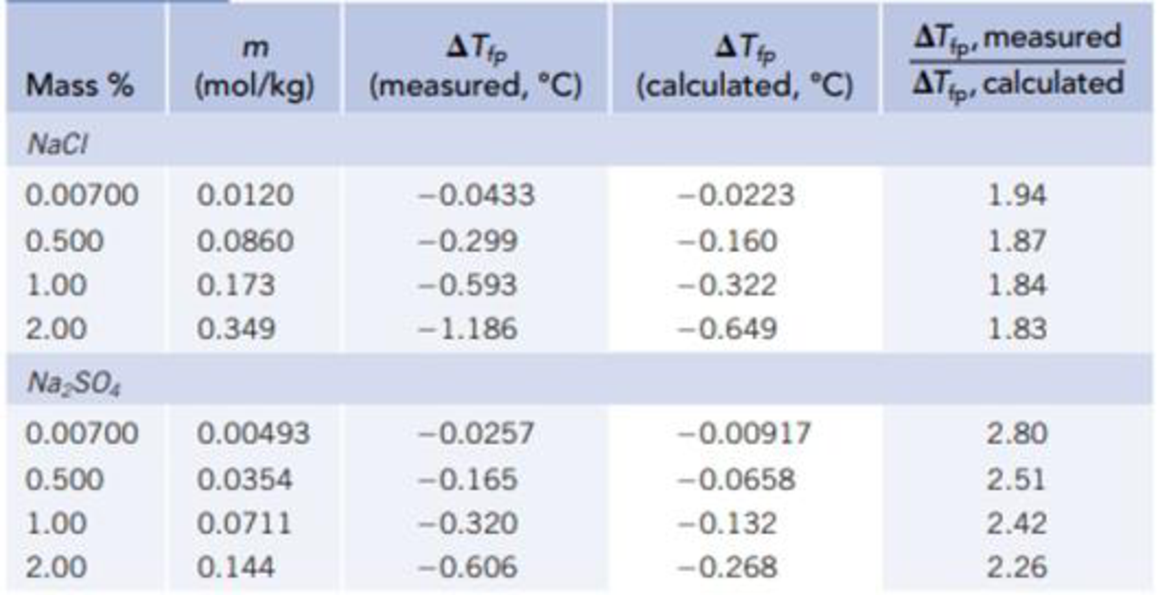Chapter 13, Problem 105SCQ

Chapter
Section
Textbook Problem

Review the trend in values of the van’t Holt factor i as a function of concentration (Table 13.4). Use the following data to calculate the van’t Hoff factor for a NaCl concentration of 5.00% (by weight) (for which ΔT = −3.05 °C) and a Na2SO4 concentration of 5.00% (by weight) (for which ΔT = −1.36 °C). Are these values in line with your expectations based on the trend in the values given in Table 13.4? Speculate on why this trend is seen.TABLE 13.4Freezing Point Depressions of Some lonic SolutionsInterpretation Introduction

Interpretation: van’t Hoff factor for NaCl and Na2SO4 have to be determined. Whether the values are following the expectations based on trend in the table 13.4 has to be identified.

Concept introduction:

Colligative properties: Properties of solutions which having influence on the concentration of the solute in it. Colligative properties are,

• Decrease in the vapor pressure
• Increase in the boiling point
• Decline in the freezing point
• Osmotic pressure

Freezing point depression: The freezing point of the solution varies with the solute concentration.

ΔTfp=Kfpmsolute

where,

Kfp is the molal freezing point depression constant.

van’t Hoff factor, i: it is the ration between change in in freezing point measured and change in in freezing point calculated. It indicates the total number of ions that are produced.

van'tHofffactor,i=measuredΔTfpCalculatedΔTfp

Molality (m): Molality is the number of moles of solute present in one kilogram of solvent.

Molality (m) =Numberofmolesofsolute1kgofsolvent

The number of moles of any substance can be determined using the equation

Numberofmole=GivenmassofthesubstanceMolarmass

Explanation

Given,

measuredΔTfpforNaCl=3.050CmeasuredΔTfpforNa2SO4=1.360CKfp=1.860C/m

Mass of NaCl is 5100×100g=5g

Molar mass of NaCl is 58.44g/mol

Mass of Na2SO4 is 5100×100g=5g

Molar mass of Na2SO4 is 142.04g/mol

Mass of water is 100g5g=95g

The number of moles of any substance can be determined using the equation

Numberofmole=GivenmassofthesubstanceMolarmass=5g58.44g/mol=0.086mol

Molarity of the NaCl solution is,

Molality (m) =NumberofmolesofsoluteSolventinkg=0.086mol0.095kg=0.90m

Molarity of the NaCl solution is 0.90m

It is assumed that dissociation does not take place.

Depression in freezing point is calculated by using the equation

ΔTfp= Kfp. msolute=(1.860C/m)(0.90m)=1.670C

Depression in freezing point when there is no dissociation is 1.670C

van'tHofffactor,i=measuredΔTfpCalculatedΔTfp

i=3

Still sussing out bartleby?

Check out a sample textbook solution.

See a sample solution

The Solution to Your Study Problems

Bartleby provides explanations to thousands of textbook problems written by our experts, many with advanced degrees!

Get Started

Find more solutions based on key concepts